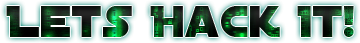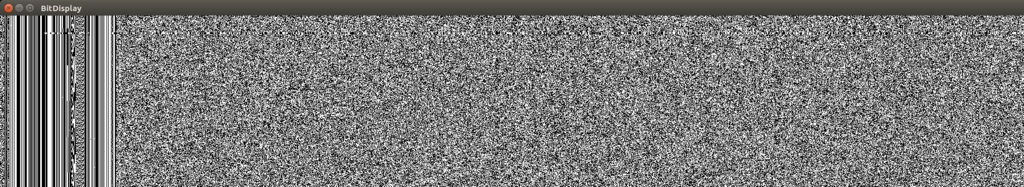# Frame Synchronization

Now we have the sync word, we can do the frame synchronization. I will use a correlation system to find the most probably location for the sync word. For that I will basically sum the difference of each XOR (UW[n] ^ data[i+n]) to a variable. The position that has the highest value (a.k.a. highest correlation) is where our word is most probably.

``````typedef struct {
uint32_t uw0mc;
uint32_t uw0p;
uint32_t uw2mc;
uint32_t uw2p;
} correlation_t ;

const uint64_t UW0 = 0xfca2b63db00d9794; // 0 degrees inverted phase shift
const uint64_t UW2 = 0x035d49c24ff2686b; // 180 degrees inverted phase shift

uint8_t UW0b; // The Encoded UW0
uint8_t UW2b; // The Encoded UW2

void initUW() {
printf("Converting Sync Words to Soft Data\n");
for (int i = 0; i < 64; i++) {
UW0b[i] = (UW0 >> (64-i-1)) & 1 ? 0xFF : 0x00;
UW2b[i] = (UW2 >> (64-i-1)) & 1 ? 0xFF : 0x00;
}
}

uint32_t hardCorrelate(uint8_t dataByte, uint8_t wordByte) {
//1 if (a        > 127 and       b == 255) or (a        < 127 and       b == 0) else 0
return (dataByte >= 127 & wordByte == 0) | (dataByte < 127 & wordByte == 255);
}

void resetCorrelation(correlation_t * corr) {
memset(corr, 0x00, sizeof(correlation_t));
}

void checkCorrelation(uint8_t *buffer, int buffLength, correlation_t *corr) {
resetCorrelation(corr);
for (int i = 0; i < buffLength - 64; i++) {
uint32_t uw0c = 0;
uint32_t uw2c = 0;

for (int k = 0; k < 64; k++) {
uw0c += hardCorrelate(buffer[i+k], UW0b[k]);
uw2c += hardCorrelate(buffer[i+k], UW2b[k]);
}

corr->uw0p = uw0c > corr->uw0mc ? i : corr->uw0p;
corr->uw2p = uw2c > corr->uw2mc ? i : corr->uw2p;

corr->uw0mc = uw0c > corr->uw0mc ? uw0c : corr->uw0mc;
corr->uw2mc = uw2c > corr->uw2mc ? uw2c : corr->uw2mc;
}
}
``````

With this piece of code, you can run checkCorrelation(buffer, length, corr) and get the correlation statistics. In the corr

object you will have the fields uw0p and uw2p with the positions of the highest correlation of the buffer and in uw0mc and uw2mc you will have the correlation of that position. With this two values you can know the position that your sync word is, and what is the phase shift that the Costas Loop locked into (if uw0 is 0 degrees, if uw2 is 180 degrees). To correct the data if the output is 180 degrees phase shifted, just invert every bit in your sequence. I do that by XOR’ing every byte with 0xFF. Now if you output this to a file and inspect with BitDisplay you will see a pattern like this:See that the syncword is pretty clear in the bit display? You can use that to visually identify sync words or static data inside the frames. You can also notice that there are even more static data in the frames. We will talk about it later. Now our data is ready to decode.